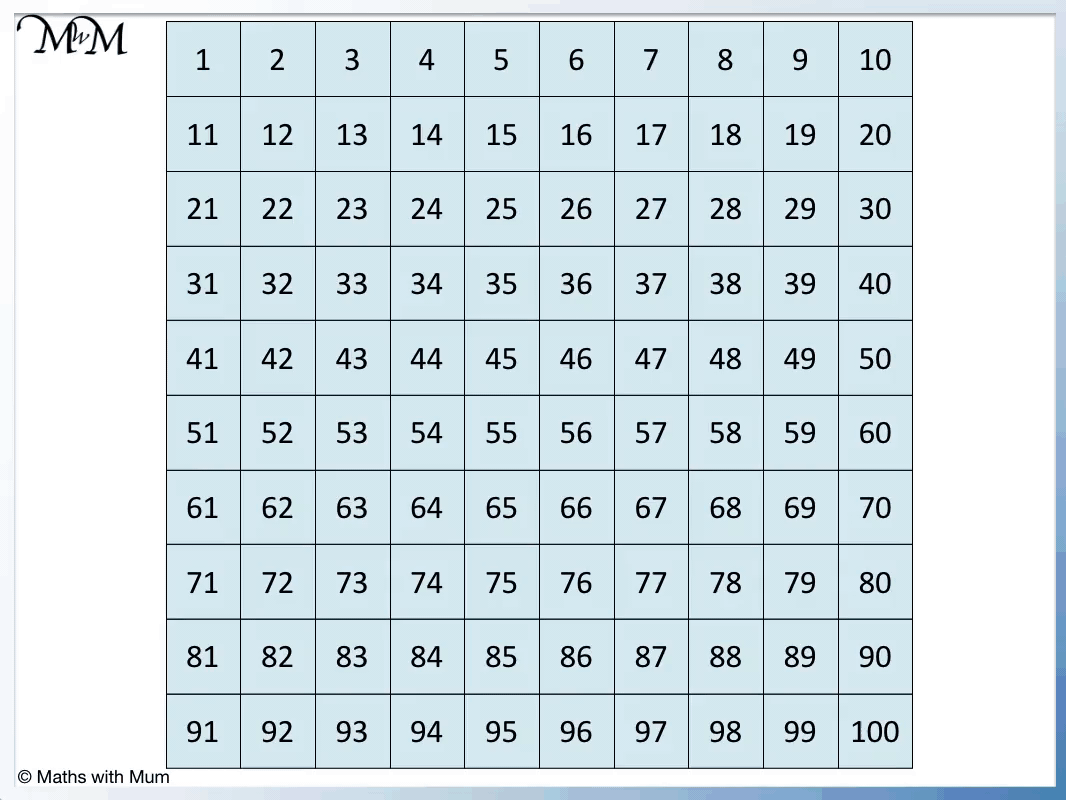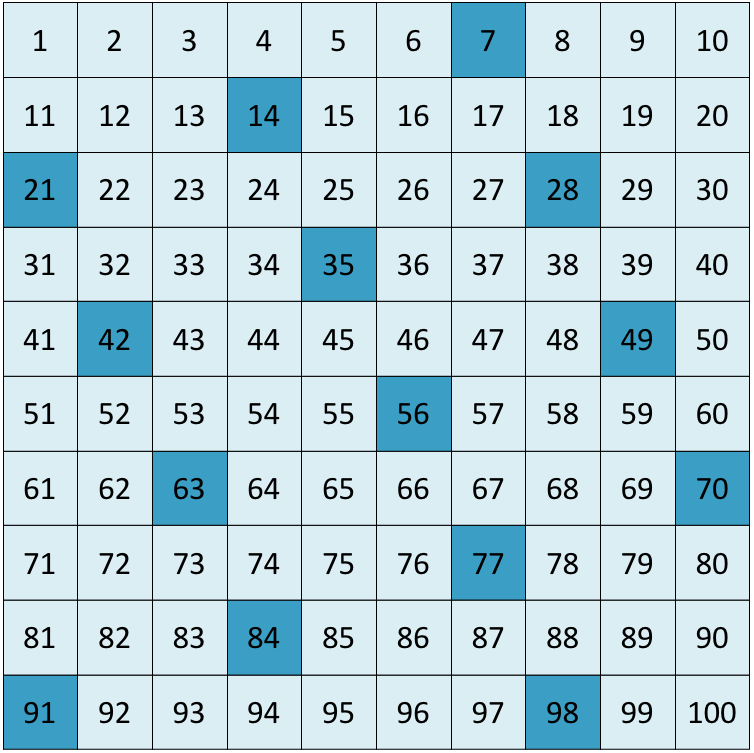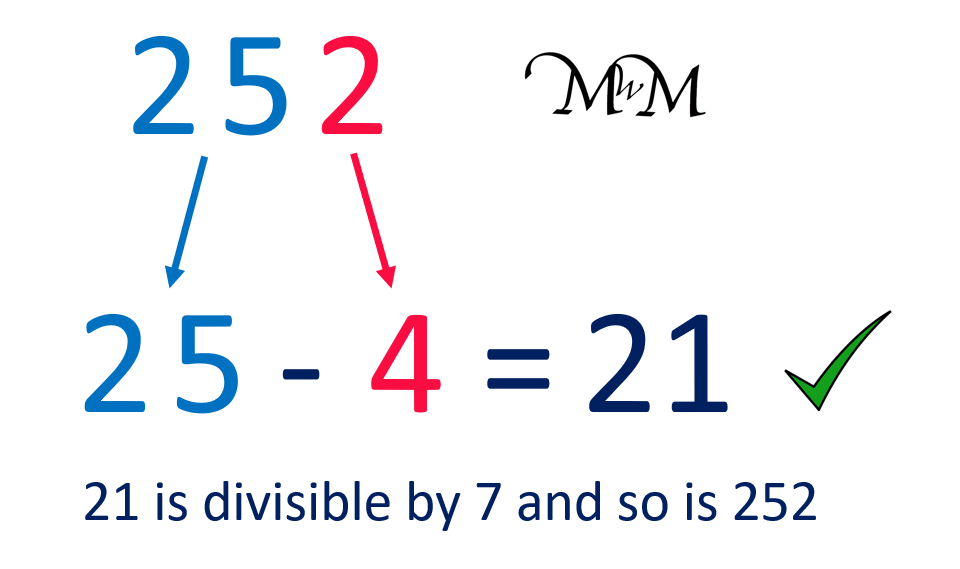# Multiples of 7

Multiples of 7• The multiples of 7 are numbers that can be divided by seven exactly, leaving no remainder.
• The answers to the seven times table are the first few multiples of 7.
• For example 3 × 7 = 21 and so, the third multiple of seven is 21.
• For example 100 × 7 = 700 and so, the hundredth multiple of seven is 700.

A multiple of 7 is any number that can be divided by seven exactly, leaving no remainder.

The first few multiples of 7 are seen in the 7 times table.Click on the multiples of 7 flashcards below to memorise the 7 of times table.

×
×

=#### Multiples of 7: Interactive Questions

Multiples of 7: Interactive Questions

# Multiples of 7

## What are Multiples of 7?

Multiples of 7 are numbers that can be divided by 7 exactly without remainder. The multiples of 7 are the numbers in the 7 times table. For example, the 100th multiple of 7 is 700 because 7 × 100 = 700.

Here are the first twelve multiples of 7 shown in the 7 times table:The first few multiples of 7 are:

• 1 × 7 = 7
• 2 × 7 = 14
• 3 × 7 = 21
• 4 × 7 = 28
• 5 × 7 = 35
• 6 × 7 = 42
• 7 × 7 = 49
• 8 × 7 = 56
• 9 × 7 = 63
• 10 × 7 = 70
• 11 × 7 = 77
• 12 × 7 = 84

There are an infinite number of multiples of 7.

Any number that can be made by multiplying by 7 is a multiple of 7.

Zero is a multiple of 7 because 0 × 7 = 0.

To find multiples of 7, start at zero and keep adding 7. If you know a multiple of 7, then simply add 7 to this number to find the next multiple of 7.

When teaching the multiples of 7, it can be helpful to place them on a number grid. A number grid is a useful teaching tool to use because it is easy to add on 7 to the previous number. Simply count on 7 squares on the grid to find the next multiple of 7.To learn the multiples of 7, it helps to identify patterns in the numbers or how the numbers are positioned on the number grid.

One way to learn some of the multiples of 7 is that the numbers 21, 42, 63 and 84 are all multiples of 7 that have a tens digit that is double the ones digit. For example, 2 is double 1 in 21 and 8 is double 4 in 84.## What are the Multiples of 7 up to 100?

There are 14 multiples of 7 that are less than 100. The first few multiples of 7 are:

7, 14, 21, 28, 35, 42, 49, 56, 63, 70, 77, 84, 91 and 98.

Here are the multiples of 7 up to 100 shown on number grid.Here is a longer list of multiples of 7:

7, 14, 21, 28, 35, 42, 49, 56, 63, 70, 77, 84, 91 and 98, 105, 112, 119, 126, 133, 140, 147, 154, 161, 168, 175, 182, 189, 196, 203, 210, 217, 224, 231, 238, 245, 252, 259, 266, 273, 280, 287, 294, 301, 308, 315, 322, 329, 336, 343, 350.

We can see that each multiple of 7 is 7 numbers away from any other multiple of 7.

## The Rule for Multiples of 7

To check if a number is a multiple of 7, follow these steps:

1. Double the last digit of the given number.
2. Remove the last digit from the given number.
3. Subtract the answer to step 1 from the answer to step 2.
4. If the result is 0 or a multiple of 7, then the original number was a multiple of 7.

For example, here is 161. 161 is a multiple of 7.To check if a number is a multiple of 7, double the last digit, subtract it from the remaining digits. If the answer is 0 or another multiple of 7, then the original number is a multiple of 7.

The last digit of 161 is 1. If we double this digit we get 2.

The remaining digits of 161 are 16.

16 – 2 = 14, which is a multiple of 7.

Since 14 is a multiple of 7, 161 is also a multiple of 7.

Here is another example. Here we have 252.The first step is to double the last digit. The last digit of 252 is 2. Double 2 is 4.

The second step is to remove the last digit. Removing the digit of 4 from 252 leaves 25.

The final step is to subtract the 4 from the 25. 25 – 4 = 21.

21 is a multiple of 7 and therefore so is 252.

Here is an example of using the rule on a number that is not a multiple of 7.

We have the number of 342.The last digit of 342 is 2. Doubling this digit gives us 4.

We subtract this 4 from the remaining digits of 34. 34 – 4 = 30.

30 is not a number in the 7 times table. 30 is not a multiple of 7 and so, 342 is not a multiple of 7 either.Now try our lesson on Lattice Multiplication Method where we learn how to use the lattice method for multiplying numbers.